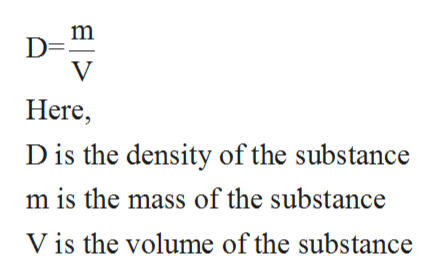# Look up the density of liquid water at 25.0 degrees C. What mass of water will occupy 250L at 25.0 degrees C? Give your answer in kg.

Question
14 views

Look up the density of liquid water at 25.0 degrees C. What mass of water will occupy 250L at 25.0 degrees C? Give your answer in kg.

check_circle

Step 1

The term density is defined as the ratio of mass to the volume of given substance. The mathematical formula is shown below:help_outlineImage TranscriptioncloseD= V Here D is the density of the substance m is the mass of the substance Vis the volume of the substance fullscreen
Step 2

The density of liquid water at 25oC is 997.0479 kg/m3.

The volume of water at 25oC is given to be 250 L.

The volume in m3 can be calculated as shown below:

Step 3

The mass of liquid water at 25oC is ca...

### Want to see the full answer?

See Solution

#### Want to see this answer and more?

Solutions are written by subject experts who are available 24/7. Questions are typically answered within 1 hour.*

See Solution
*Response times may vary by subject and question.
Tagged in

### Physical Chemistry